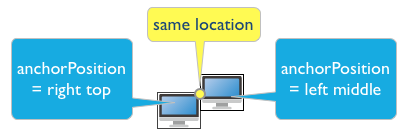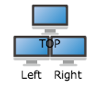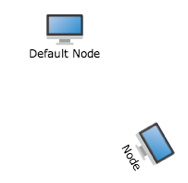Light High Efficiency Graph Component

# Node Position

## Property of node position

• #location - Coordinate position
• #rotate - Rotate angle
• #zIndex - Layer property for controlling the display layer of graph. The high value one is displayed at the upper layer
• #anchorPosition - Anchor position, which can control the align method of nodes. The same position coordinate and different anchors will present different effects. It is defaulted to be the center of node graph

### Anchor position#### Example

The node at same position and different anchors

``````var node = graph.createNode("Right");
node.anchorPosition = Q.Position.LEFT_TOP;
node = graph.createNode("Left");
node.anchorPosition = Q.Position.RIGHT_TOP;
node = graph.createNode("TOP");
node.anchorPosition = Q.Position.CENTER_BOTTOM;
``````

Operation effect:#### Example

Create nodes, and set appointed position, rotate angle and anchor position

``````var defaultNode = graph.createNode("Default Node");
var node = graph.createNode("Node");
node.location = new Q.Point(100, 100);
node.rotate = Math.PI / 3;
node.anchorPosition = Q.Position.LEFT_TOP;
``````

Operation effect: